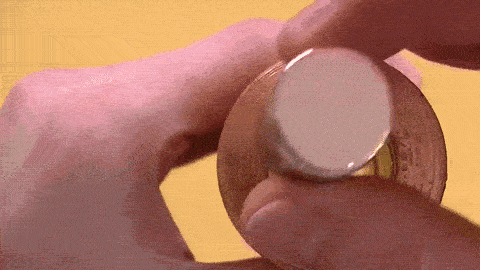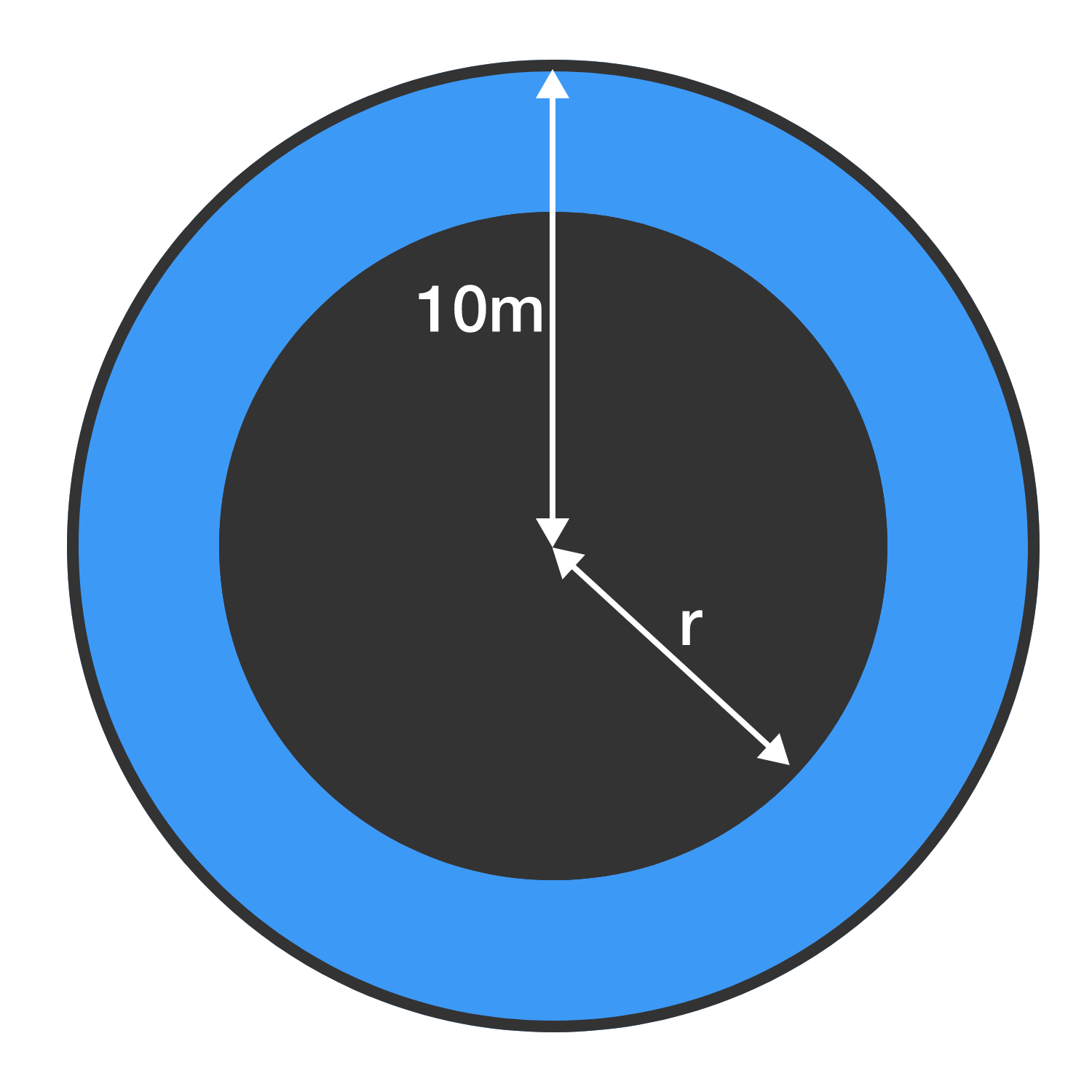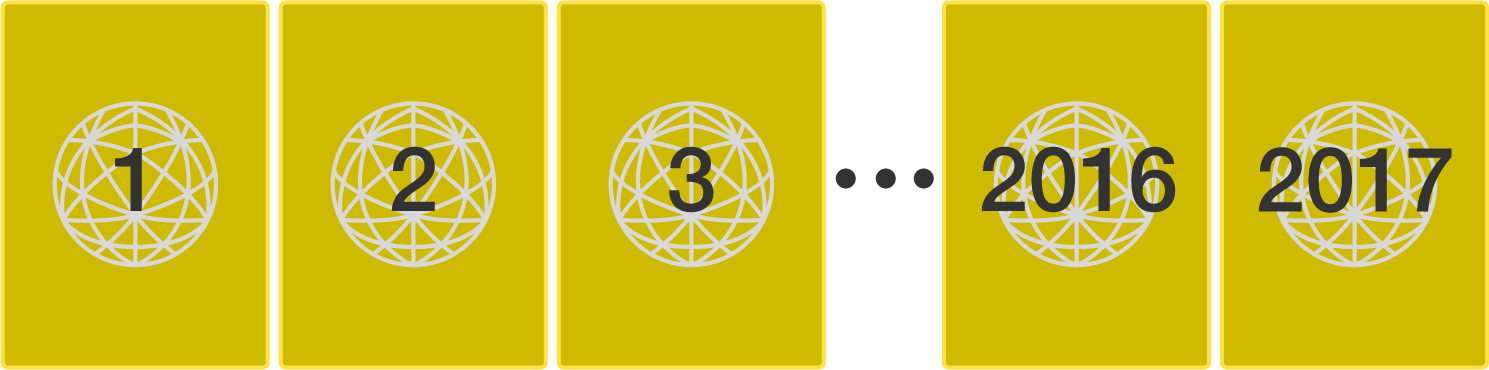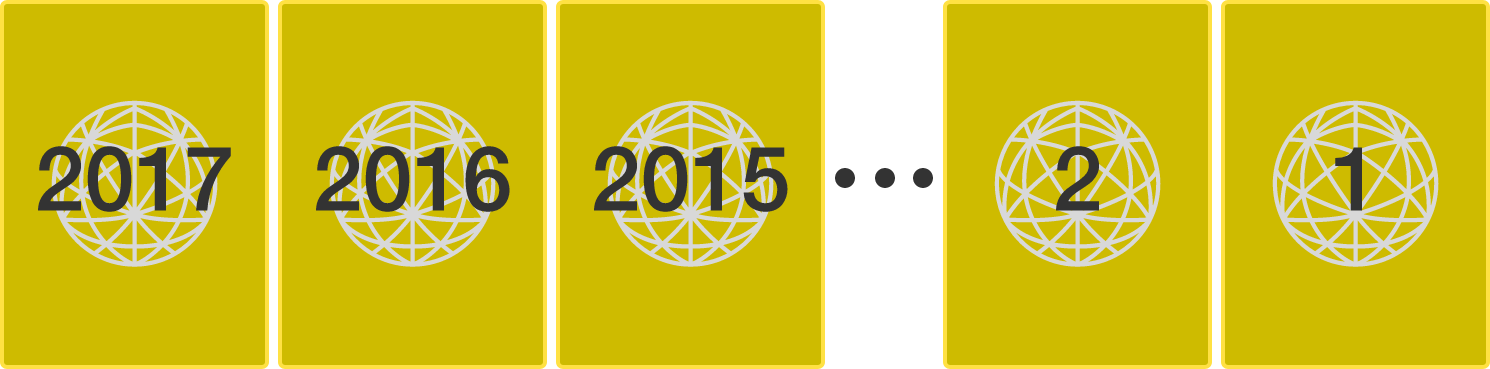# Problems of the Week

Contribute a problem

# 2017-03-13 IntermediateThe above video shows a copper tube in which a magnet is dropped. Why does the magnet fall so slowly in the copper tube?

$\frac{1}{1}+\frac{1}{1+2}+\frac{1}{1+2+3}+\cdots$

Find the value of the sum to 2 decimal places.An art museum wants to build a new gallery. The floor plan is an annulus (a ring shape bounded by two concentric circles), with walls constructed on both circles. The outer circle has a radius of $10\text{ m}$, and we are not able to see through the inner circle.

The museum will hire guards to ensure that the public does not touch the artwork. Each guard will stand at a fixed spot, but can look in all directions from that spot.

If they can afford to hire only 4 guards, and every part of the gallery must be visible to at least one guard, then what is the maximum (supremum) possible radius of the inner circle in meters?

The integers from 1 to 1000 (inclusive) are to be placed into boxes such that no box contains a number that is a multiple of another number in the same box.

What is the minimum number of boxes needed?

As an explicit example, the integers from 1 to 10 can be placed into 4 boxes while satisfying the condition:

• Box 1 - $\{ 1 \}$
• Box 2 - $\{ 2, 3, 5, 7 \}$
• Box 3 - $\{ 4, 6, 9 , 10\}$
• Box 4 - $\{ 8 \}$You have 2017 cards numbered $1, 2, 3, \ldots, 2017$, as shown above. In each move, you can swap two adjacent cards.

What is the minimum number of moves required for the above cards to be arranged backwards as $2017, 2016, \ldots, 2, 1$?As an explicit example, if you started with four cards arranged as 1234, in one move you could go to 2134, 1324, or 1243 only.

×